# relativistic quantum theory

Also found in: Dictionary, Thesaurus.

## Relativistic quantum theory

The quantum theory of particles which is consistent with the special theory of relativity, and thus can describe particles moving arbitrarily close to the speed of light. It is now realized that the only satisfactory rela-tivistic quantum theory is quantum field theory; the attempt to relativize the Schrödinger equation for the wave function of a single particle fails [Eq. (1)]. However, with a change of interpretation, relativistic wave equations do correctly describe some aspects of the motions of particles in an electromagnetic field. See Quantum field theory, Quantum mechanics, Relativity

The Schrödinger equation for the wave function ψ( r ,t) of a particle is

(1)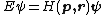Eq. (1), where E is the energy operator , p is the momentum operator -i&planck;Δ, H( p , r ) is the classical hamiltonian, and &planck; is Planck's constant divided by 2π. For a nonrelativistic free particle, H = p 2/2m. The naive way to relativize Eq. (1) would be to use the relativistic hamiltonian, Eq. (2). However, this equation is not relativistically
(2)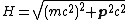invariant.

The so-called Klein-Gordon equation (3)

(3)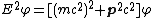is relativistically invariant. However, the only possible density of a conserved quantity formed from &phiv; is of the form shown in (4). But this cannot be a probability density, because it is not positive definite (it changes sign when &phiv; is replaced by &phiv;*).
(4)But ρ, in relation (4), can be interpreted as a charge density (when multiplied by a unit charge e); &phiv; is then to be interpreted as a matrix element of a field operator &PHgr; of a quantized field whose quanta are particles with mass m and charge e or -e and zero spin.

P. A. M. Dirac found a relativized form of Eq. (1), Eq. (5), which is both linear in E and has a positive definite density form ρ, where β and α are constants which obey Eqs. (6).

(5)(6)Obviously the four constants β and αi cannot be numbers; however, they can be 4 × 4 matrices, and Ψ is then a four-component object called a Dirac spinor.

If plane wave solutions of Dirac's equation (5) are considered, then P is now a number. Taking Eq. (5) as an eigenequation for E, four eigenstates are found (because H is a 4 × 4 matrix): two with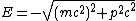and two with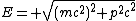The interpretation of the two positive energy states is that they are the two spin states of a particle with spin ½[&planck;]. But the two negative energy states are an embarrassment; even a particle that was initially in a positive energy state would quickly make radiative transitions down through the negative energy states. Dirac's solution was to observe that if the particle described by ψ obeyed the Pauli principle, then one can suppose that all the negative energy states are already filled with particles, thus excluding any more. There are still four single-particle states for a given momentum p : the two spin states of a particle with positive energy, and the two states obtained by removing a negative energy particle (of momentum - p ). These last states (“hole states”) have positive energy and a charge opposite the charge of the particle. The hole is in fact the antiparticle; if the particle is an electron, the hole is a positron. See Antimatter, Positron

With the filling up of the negative energy states, one no longer has a single-particle system, and ψ, just as in the Klein-Gordon case, no longer can be interpreted as a wave function but must be interpreted as a matrix element of a field operator Ψ.

McGraw-Hill Concise Encyclopedia of Physics. © 2002 by The McGraw-Hill Companies, Inc.

## relativistic quantum theory

[‚rel·ə·tə′vis·tik ′kwänt·əm ‚thē·ə·rē]
(quantum mechanics)
The quantum theory of particles which is consistent with the special theory of relativity, and thus can describe particles moving arbitrarily close to the speed of light.
McGraw-Hill Dictionary of Scientific & Technical Terms, 6E, Copyright © 2003 by The McGraw-Hill Companies, Inc.
References in periodicals archive ?
The new high-temperature form of superconductivitythat is currently setting the physics world on its ear may also illustrate the problems of applying quantum mechanics to small numbers of objects, Edward Teller of Lawrence Livermore (Calif.) National Laboratory told the Loyola Conference on Mathematical and Interpretational Problems in Relativistic Quantum Theory, meeting in New Orleans last week.
However, putting Lorentz and Heisenberg algebra together should have given us a stable, relativistic quantum theory but unfortunately it did not.
If this claim is correct then the variational principle is also a necessary condition for constructing a self-consistent relativistic quantum theory.

Site: Follow: Share:
Open / Close